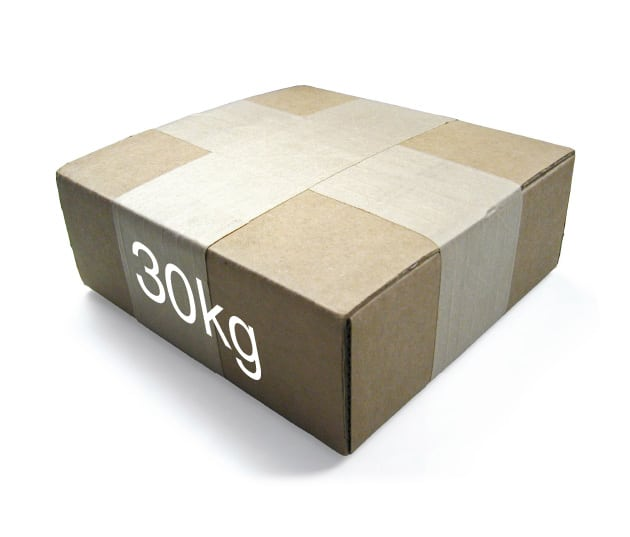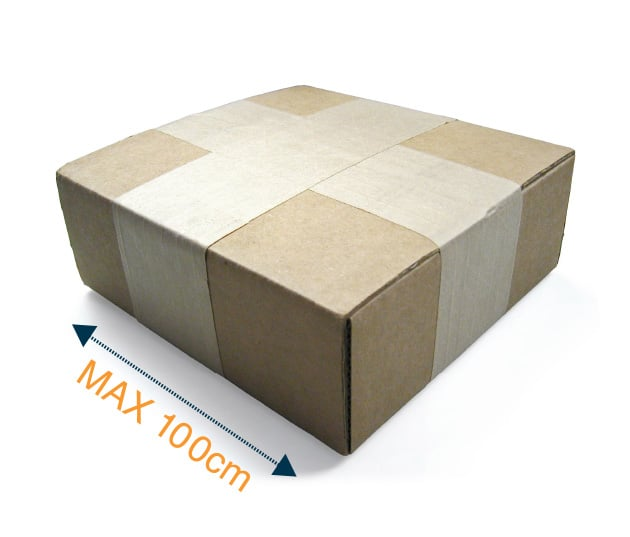# Weights & DimensionsLet us know what can we do for you. Contact us today!## WEIGHTS & DIMENSIONS

Here you’ll find some guidance on size and weight limitations when sending your parcel. Do remember that all carriers calculate what is known as a “Volumetric Weight” and your parcel delivery rate will be for either actual or volumetric weight whichever is the greater.## Recommended Maximum Package Weight – 30kg

For anything over this weight, the driver may need assistance with handling## Maximum Length – 100cm

The maximum acceptable length of a parcel is 100cm.## Volumetric Weight

Sometimes a parcel isn’t very heavy but is still quite big. If this is this case, we work out the parcel’s “volumetric weight”. We calculate this by taking the volume of the parcel and dividing it by 5000 i.e.

Length x width x height5000

= 14kg

So if you have a 5kg parcel that is 100cm long, 35cm wide and 20cm high, the volumetric weight would be:

100 x 35 x 205000

= 14kg## Maximum size – 300cm

The maximum acceptable length and girth of a parcel is 300cm.

This is calculated by:

1. Adding together the 2 shortest sides
2. Multiplying by 2
3. Then adding the longest side

For example:

1. 35 + 20 = 55
2. 55 x 2 = 110
3. 110 + 100 = 210cm## Chargable Weight Calculator

Use this tool to calculate your volumetric weight and overall size to confirm what your chargeable weight will be.